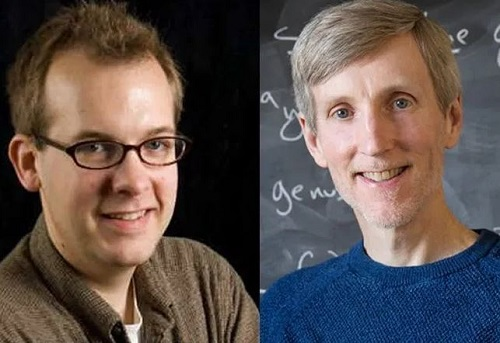# Mathematicians Deploy 500,000 Computers, One Million Hours To Solve 64-year-old Maths Riddle

 TonyGists App 3 Out Now. Download from Play store https://play.google.com/store/apps/details?id=com.TonyGists.TonyGistsapp A team led by the University of Bristol and Massachusetts Institute of Technology (MIT) has solved the final piece of the famous 65-year-old mathematics puzzle.The Mathematicians who solved the age-long mathematical puzzle     Two mathematicians have used a global network of 500,000 computers to solve a centuries-old math puzzle.They spent a million hours working on the equation before arriving at the answer.The question, which goes back to at least 1955 and may have been pondered by Greek thinkers as early as the third century AD, asks, “How can you express every number between 1 and 100 as the sum of three cubes?” Or, put algebraically, how do you solve x^3 + y^3 + z^3 = k, where k equals any whole number from 1 to 100?   As reported by Live Science, “This deceptively simple stumper is known as a Diophantine equation.”It was named for the ancient mathematician Diophantus of Alexandria, who proposed a similar set of problems about 1,800 years ago.Live Science stated that modern mathematicians who revisited the puzzle in the 1950s quickly found solutions when ‘K’ equals many of the smaller numbers, but a few, particularly stubborn integers, soon emerged.The two trickiest numbers, which still had outstanding solutions by the beginning of 2019, were 33 and 42, the medium stated.“In April, mathematician Andrew Booker, of the University of Bristol in England, knocked 33 off the list.“Using a computer algorithm to look for solutions to the Diophantine equation with x, y and z values that included every number between positive and negative 99 quadrillion, Booker found the solution to 33 after several weeks of computing time.“Still, this exhaustive search turned up no solutions for 42, suggesting that, if there was an answer, some of the integers must be greater than 99 quadrillion.“Calculating values that large would take an insane amount of computing power; so, for his next attempt, Booker enlisted the help of Massachusetts Institute of Technology mathematician Andrew Sutherland, who helped Booker book some time with a worldwide computer network called Charity Engine.According to a statement from the University of Bristol, this network is a “worldwide computer” that borrows idle computing power from more than 500,000 home PCs around the globe.“Using this crowd-sourced supercomputer and one million hours of processing time, Booker and Sutherland finally found an answer to the Diophantine equation where K equals 42.And so, without further ado, the question and answer to the meaning of life, the universe and everything is:(-80538738812075974)^3 + (80435758145817515)^3 + (12602123297335631)^3 = 42.

Are You On youtube? subscribe to Xtremely Honest Channel Latest Updates - @Xtremely Honest

Are You On FACEBOOK? Like Our Page For Latest Updates - tonygists

Previous
Next Post »